Скачать презентацию Introduction to Matlab Patrice Koehl Department of Biological

a911ab738f5684ba7f714eca69d95f51.ppt

• Количество слайдов: 27Introduction to Matlab Patrice Koehl Department of Biological Sciences National University of Singapore http: //www. cs. ucdavis. edu/~koehl/Teaching/BL 5229 [email protected] edu. sgWhat is MATLAB? Ø A high-performance language for technical computing (Mathworks, 1998) Ø The name is derived from MATrix Laboratory Ø Typical uses of MATLAB Mathematical computations Algorithmic development Model prototyping Data analysis and exploration of data (visualization) Scientific and engineering graphics for presentation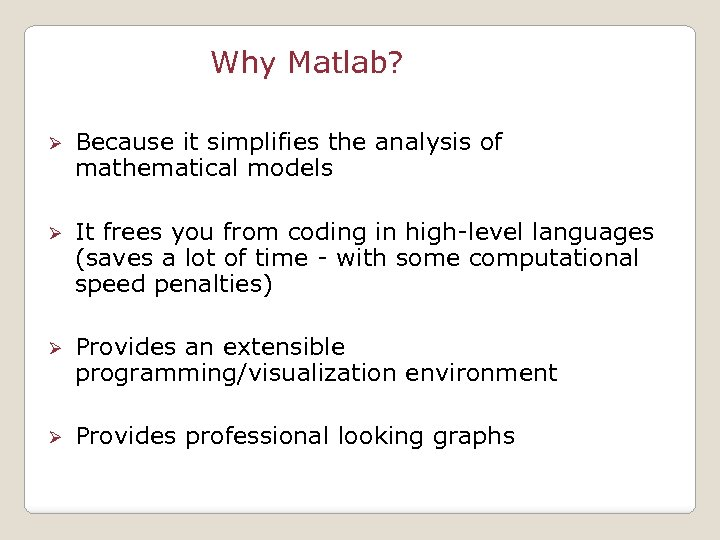Why Matlab? Ø Because it simplifies the analysis of mathematical models Ø It frees you from coding in high-level languages (saves a lot of time - with some computational speed penalties) Ø Provides an extensible programming/visualization environment Ø Provides professional looking graphs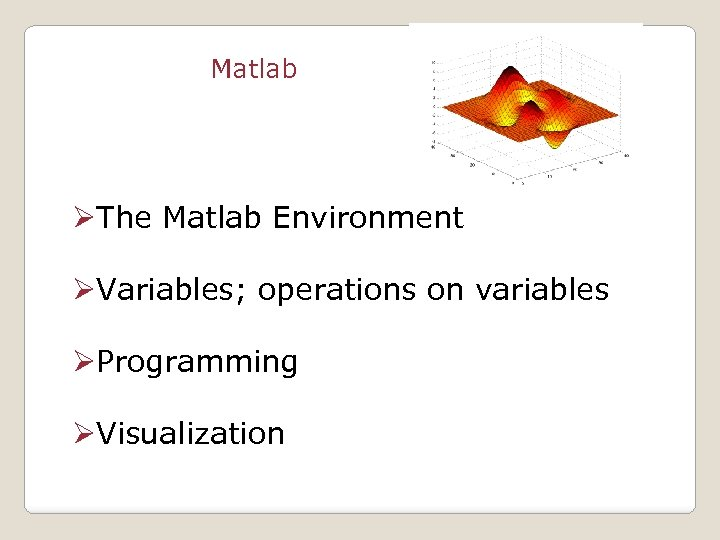Matlab ØThe Matlab Environment ØVariables; operations on variables ØProgramming ØVisualization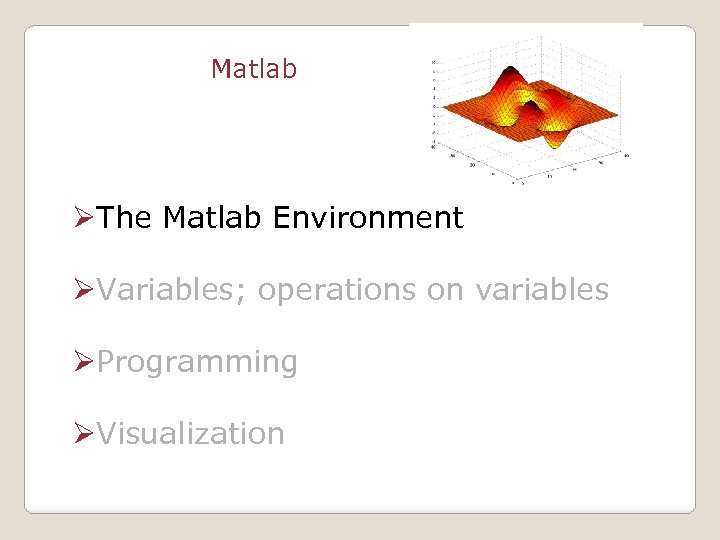Matlab ØThe Matlab Environment ØVariables; operations on variables ØProgramming ØVisualization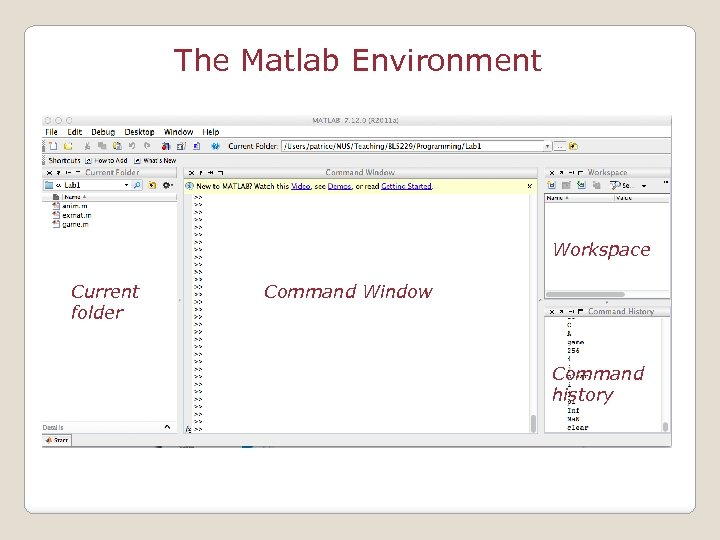The Matlab Environment Workspace Current folder Command Window Command history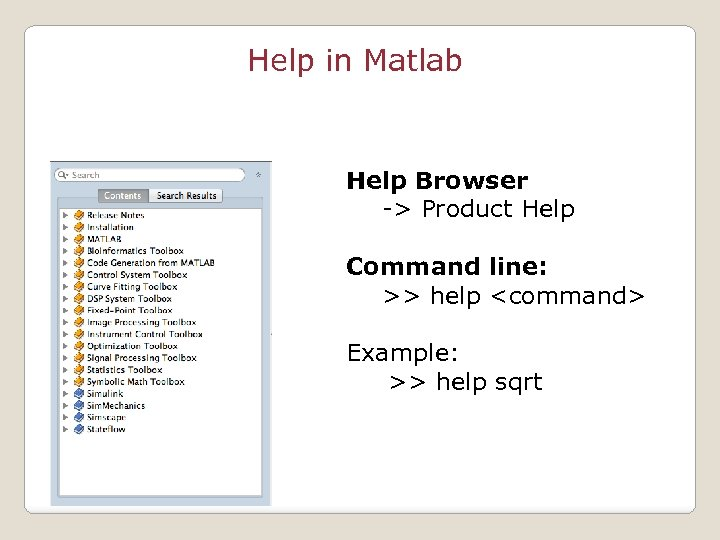Help in Matlab Help Browser -> Product Help Command line: >> help Example: >> help sqrt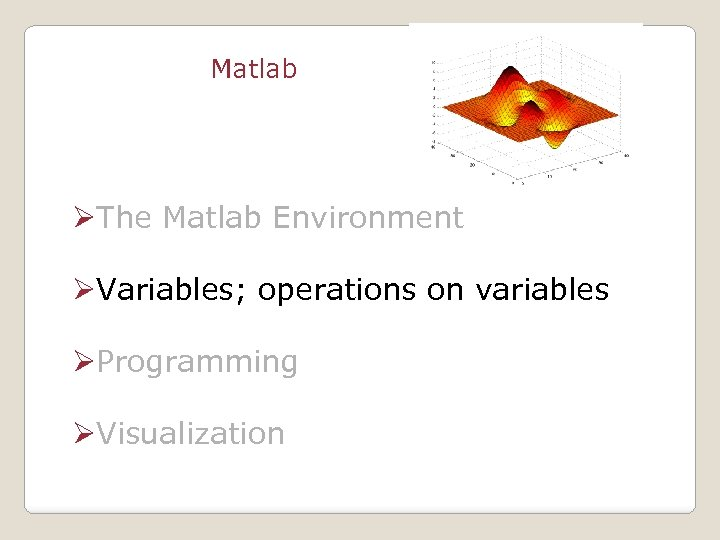Matlab ØThe Matlab Environment ØVariables; operations on variables ØProgramming ØVisualization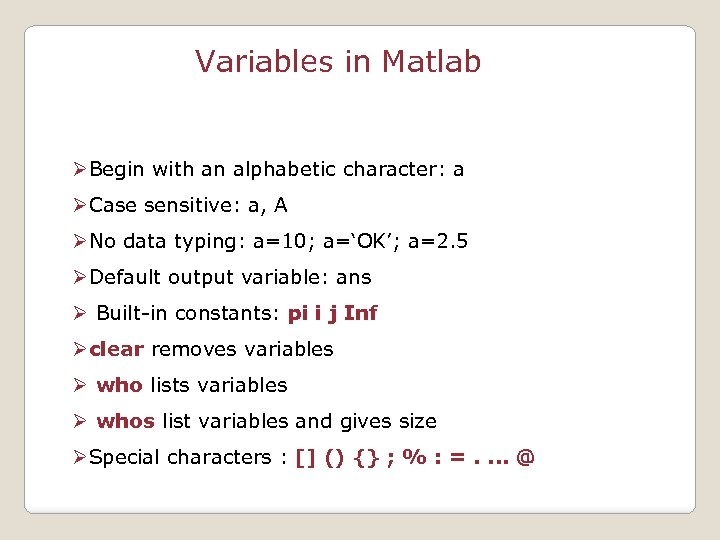Variables in Matlab ØBegin with an alphabetic character: a ØCase sensitive: a, A ØNo data typing: a=10; a=‘OK’; a=2. 5 ØDefault output variable: ans Ø Built-in constants: pi i j Inf Øclear removes variables Ø who lists variables Ø whos list variables and gives size ØSpecial characters : [] () {} ; % : =. . @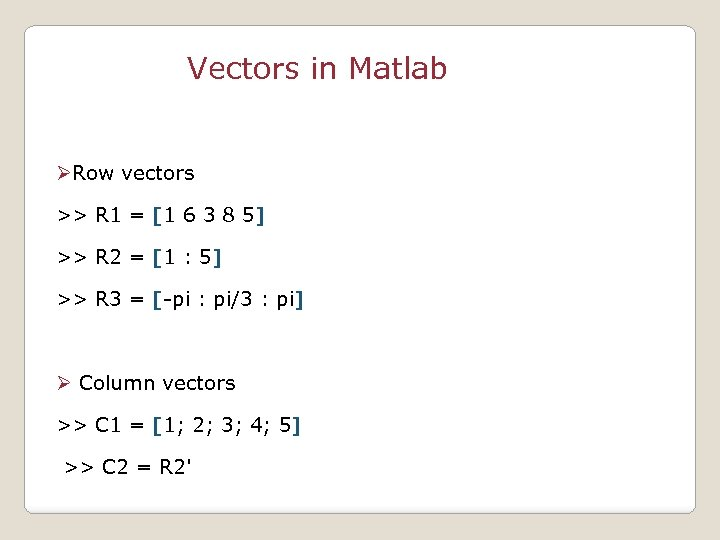Vectors in Matlab ØRow vectors >> R 1 = [1 6 3 8 5] >> R 2 = [1 : 5] >> R 3 = [-pi : pi/3 : pi] Ø Column vectors >> C 1 = [1; 2; 3; 4; 5] >> C 2 = R 2'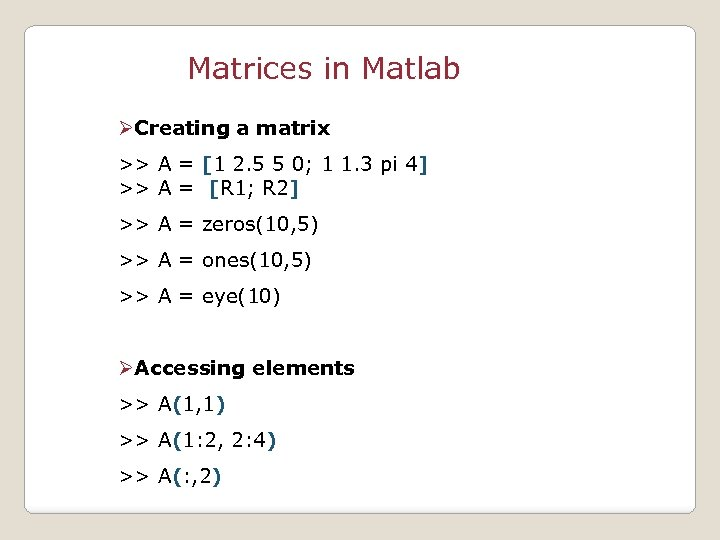Matrices in Matlab ØCreating a matrix >> A = [1 2. 5 5 0; 1 1. 3 pi 4] >> A = [R 1; R 2] >> A = zeros(10, 5) >> A = ones(10, 5) >> A = eye(10) ØAccessing elements >> A(1, 1) >> A(1: 2, 2: 4) >> A(: , 2)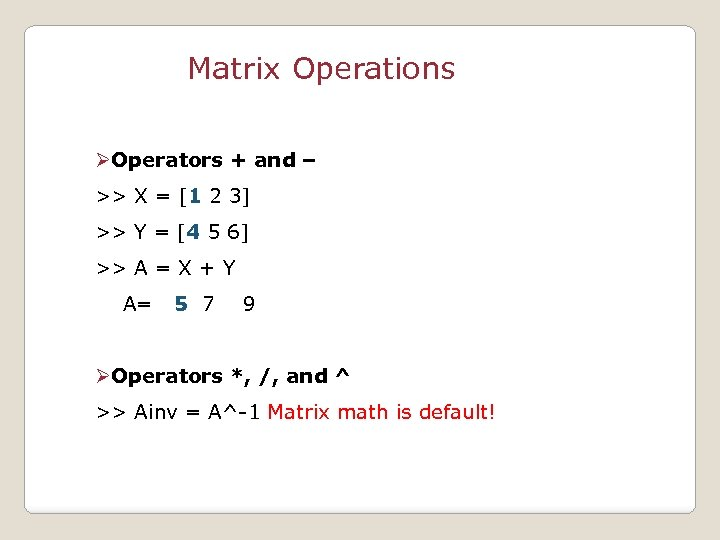Matrix Operations ØOperators + and – >> X = [1 2 3] >> Y = [4 5 6] >> A = X + Y A= 5 7 9 ØOperators *, /, and ^ >> Ainv = A^-1 Matrix math is default!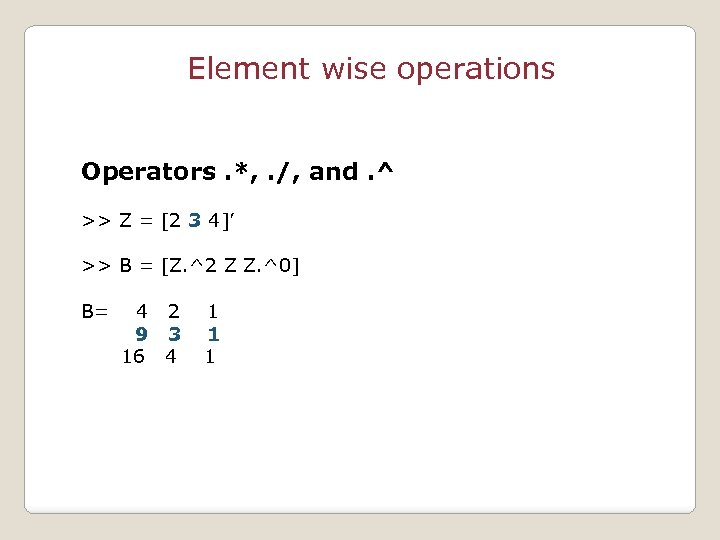Element wise operations Operators. *, . /, and. ^ >> Z = [2 3 4]’ >> B = [Z. ^2 Z Z. ^0] B= 4 2 9 3 16 4 1 1 1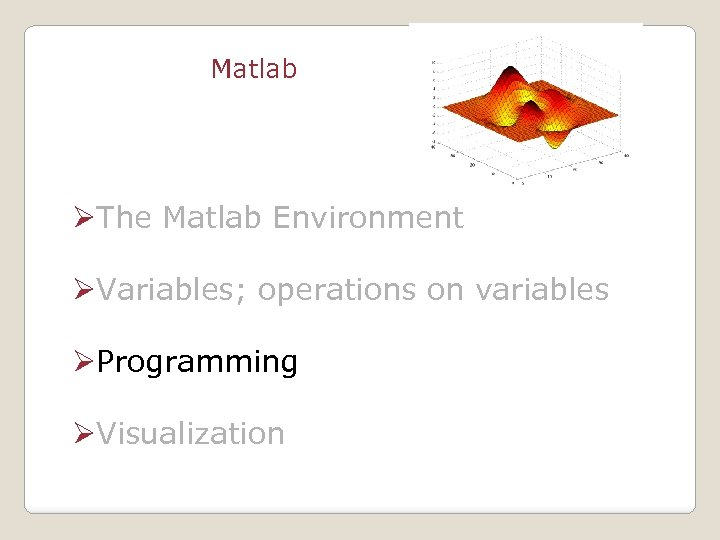Matlab ØThe Matlab Environment ØVariables; operations on variables ØProgramming ØVisualization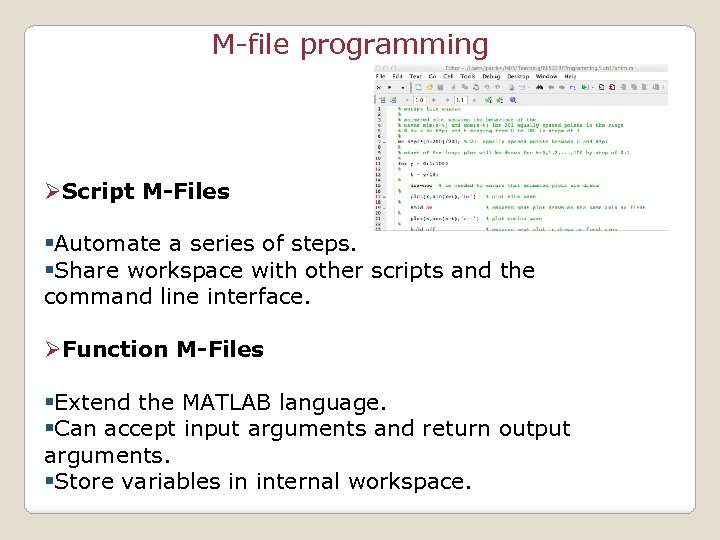M-file programming ØScript M-Files §Automate a series of steps. §Share workspace with other scripts and the command line interface. ØFunction M-Files §Extend the MATLAB language. §Can accept input arguments and return output arguments. §Store variables in internal workspace.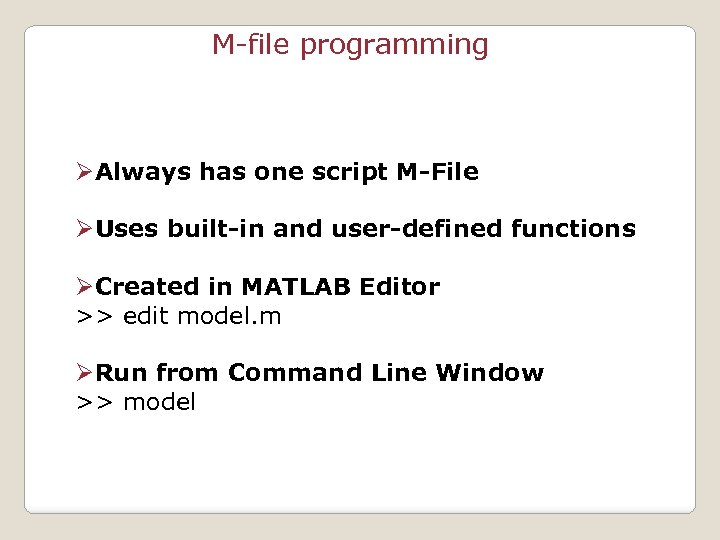M-file programming ØAlways has one script M-File ØUses built-in and user-defined functions ØCreated in MATLAB Editor >> edit model. m ØRun from Command Line Window >> model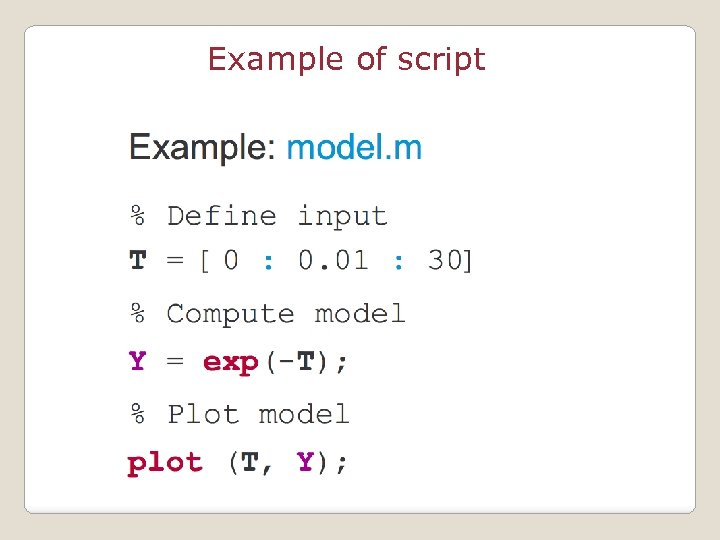Example of script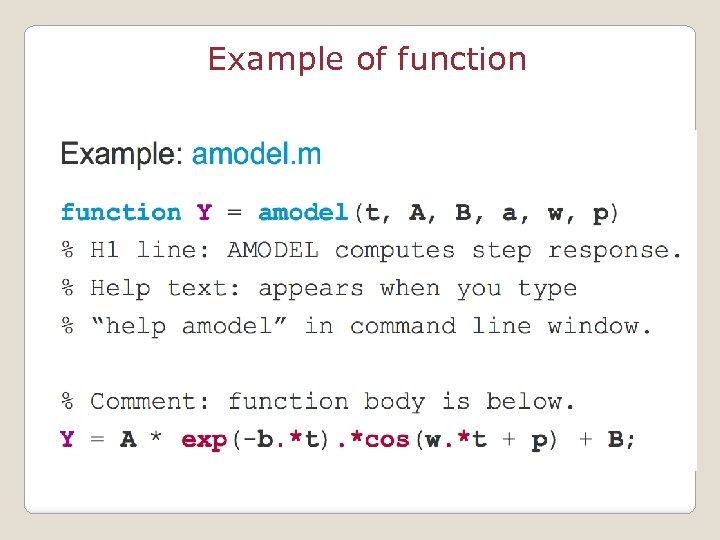Example of function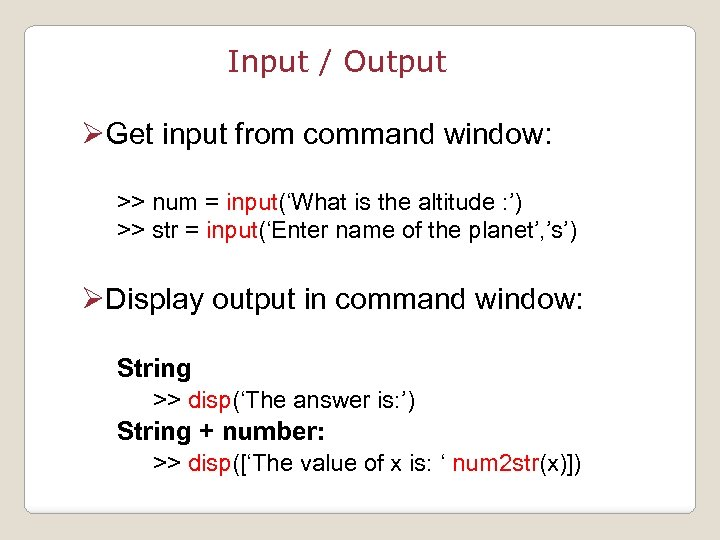Input / Output ØGet input from command window: >> num = input(‘What is the altitude : ’) >> str = input(‘Enter name of the planet’, ’s’) ØDisplay output in command window: String >> disp(‘The answer is: ’) String + number: >> disp([‘The value of x is: ‘ num 2 str(x)])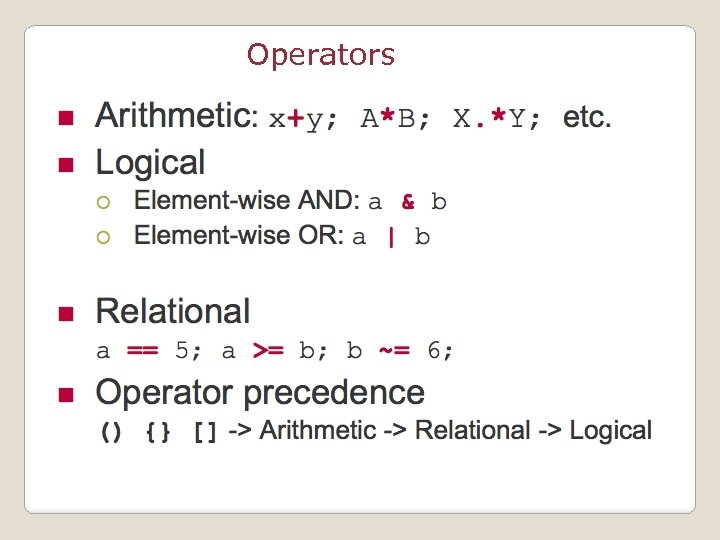Operators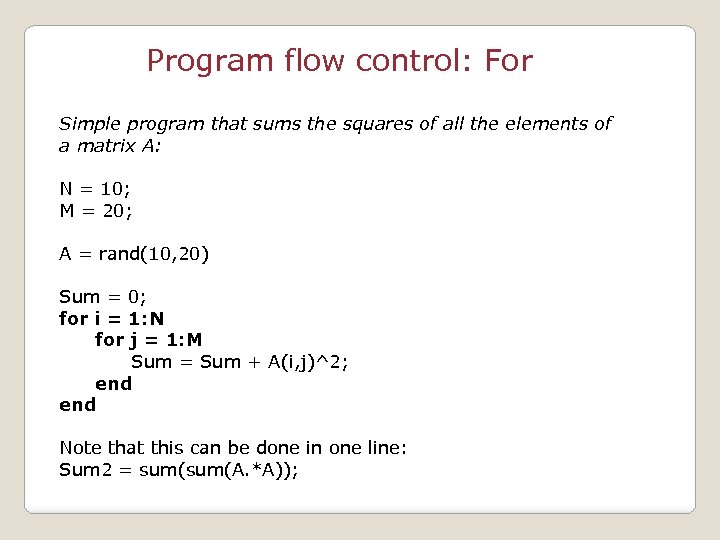Program flow control: For Simple program that sums the squares of all the elements of a matrix A: N = 10; M = 20; A = rand(10, 20) Sum = 0; for i = 1: N for j = 1: M Sum = Sum + A(i, j)^2; end Note that this can be done in one line: Sum 2 = sum(A. *A));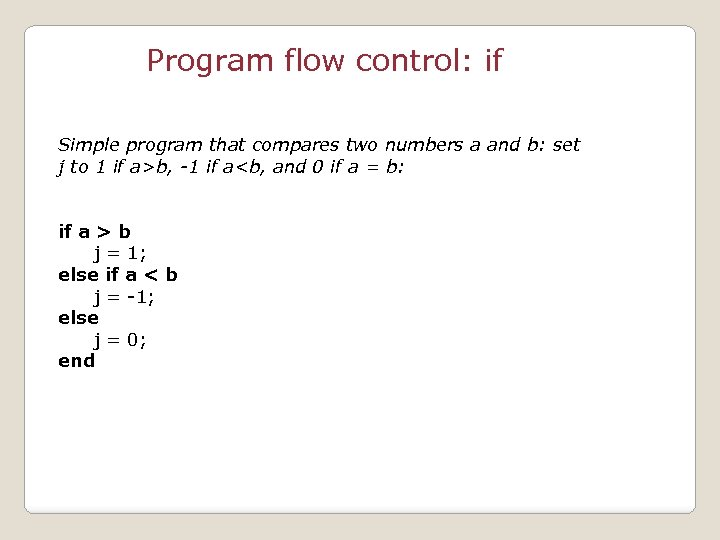Program flow control: if Simple program that compares two numbers a and b: set j to 1 if a>b, -1 if a b j = 1; else if a < b j = -1; else j = 0; end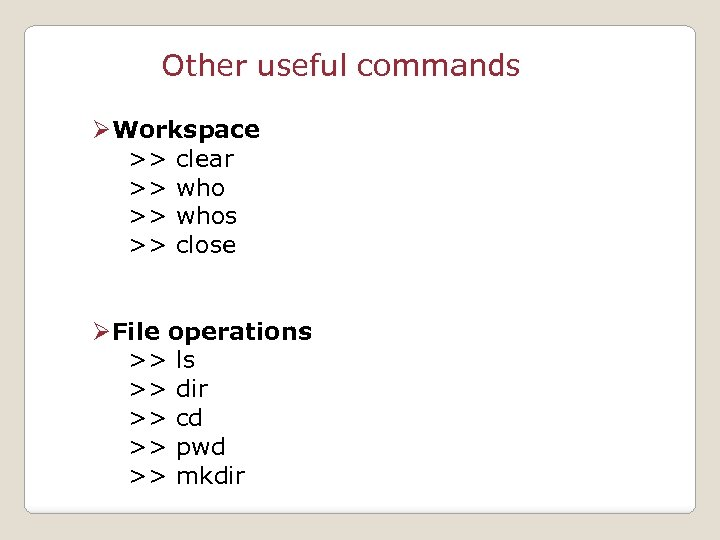Other useful commands ØWorkspace >> clear >> whos >> close ØFile operations >> ls >> dir >> cd >> pwd >> mkdir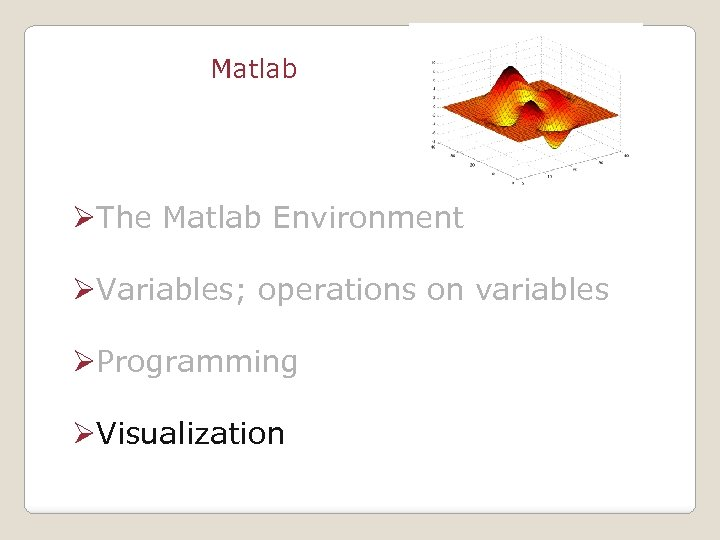Matlab ØThe Matlab Environment ØVariables; operations on variables ØProgramming ØVisualization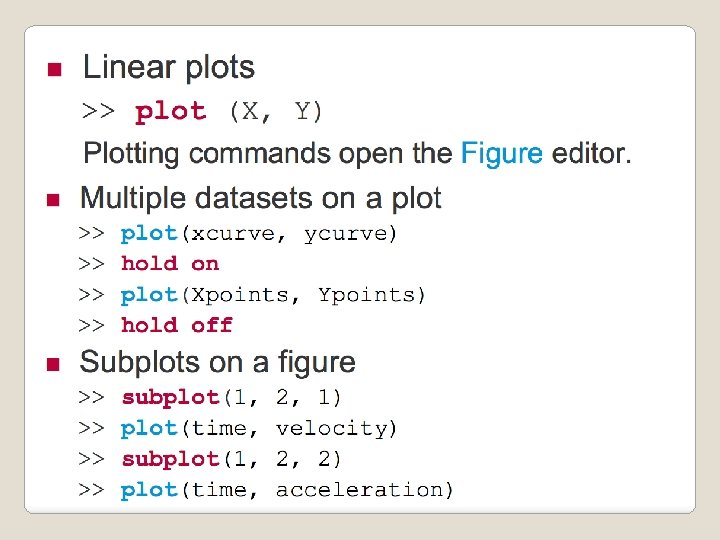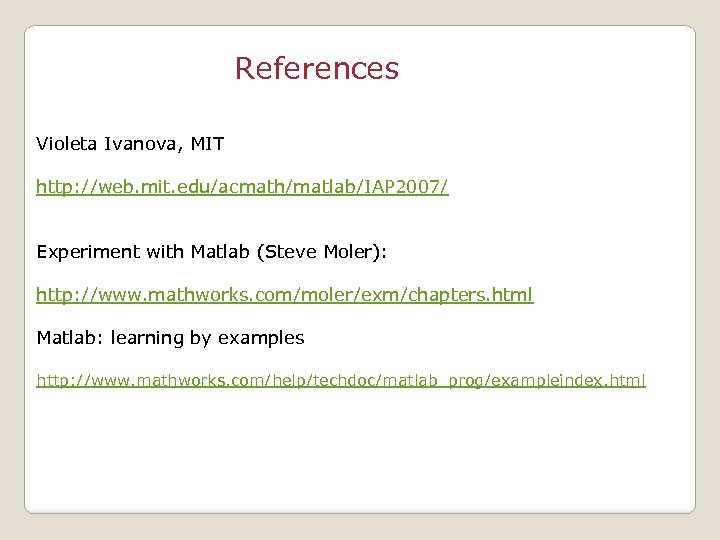References Violeta Ivanova, MIT http: //web. mit. edu/acmath/matlab/IAP 2007/ Experiment with Matlab (Steve Moler): http: //www. mathworks. com/moler/exm/chapters. html Matlab: learning by examples http: //www. mathworks. com/help/techdoc/matlab_prog/exampleindex. html